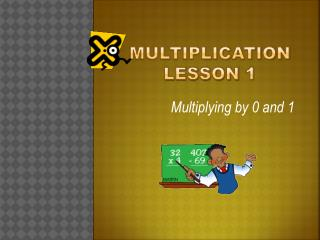# Multiplication lesson 1 - PowerPoint PPT PresentationDownload PresentationMultiplication lesson 1

Multiplication lesson 1Download Presentation## Multiplication lesson 1

- - - - - - - - - - - - - - - - - - - - - - - - - - - E N D - - - - - - - - - - - - - - - - - - - - - - - - - - -
##### Presentation Transcript

1. Multiplication lesson 1 Multiplying by 0 and 1

2. Multiplication vocabulary • Before we learn how to multiply, there are some important vocabulary words to know and understand... • Multiplication: An operation that gives the totalnumber when you join equalgroups. Example: 4 + 4 + 4 + 4 = 16 or 4 x 4 = 16 • Factors: Numbers that are multiplied to give a product. Example: 3 x 2 = 6 (3 and 2 are factors) • Product: The answer to a multiplicationproblem. Example: 5 x 2 = 10 (10 is the product)

3. Multiplication vocabulary continued • Array: An array is a set of objects or numbers arrangedinorder, often in rows & columns. Example:

4. Multiplication Factsare easiest to learn when... • You find patterns. Example: 2 x 7 = 14 can be found by counting by twos 2, 4, 6, 8, 10, 12, 14 • You use rhymes. Example: 7 and 7 are doing fine, 7 times 7 is 49; 8 and 4 were sad and blue, 8 times 4 is 32 • You use stories. Example: 7’s Tall Stories http://illuminations.nctm.org/Lessons/3-5/times6times7/SevensTallTales.mp3 • You use songs: Example: School House Rock Multiplication Rock http://www.schoolhouserock.tv/Multiplication.html

5. How to multiply by zero (0) • Rule: Any number times zero is alwayszero! Zero Times Table • 0 x 0 = 0 • 0 x 1 = 0 1 x 0 = 0 • 0 x 2 = 0 2 x 0 = 0 • 0 x 3 = 0 3 x 0 = 0 • 0 x 4 = 0 4 x 0 = 0 • 0 x 5 = 0 5 x 0 = 0 • 0 x 6 = 0 6 x 0 = 0 • 0 x 2 365 778 = 0

6. 0 x 7 = 3 x 0 = 5 x 0 = 0 x 9 = 0 x 6 = 0 x 0 = 4 x 0 = 1 x 0 = 0 0 0 0 0 0 0 0 Now let’s practice the zero times tablesGet your pencil ready and answer the following questions

7. HOW TO MULTIPLY BY ONE (1) • Rule: One times any number is the samenumber • Groups: There is only one group Example: 1 x 5 = 5, one group of 5 is 5 • Arrays: 3 rows of 1 is 3 1 row of 3 is 3 Example: 3 x 1 = 3 1 x 3 = 3

8. 5 x 1 = 3 x 1 = 1 x 1 = 1 x 7 = 1 x 0 = 12 x 1 = 6 x 1= 1 x 4 = 5 3 1 7 0 12 6 4 Now let’s practice the one times tablesGet your pencil ready and answer the following questions